### Home > CC4 > Chapter 8 > Lesson 8.2.1 > Problem8-101

8-101.
1.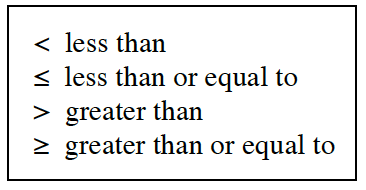Review the meanings of the inequality symbols in the box at right. Then decide if the statements below are true or false. Homework Help ✎

2.  a 5 < 7 b. −2 ≥ 9 c. 0 ≤ 0 d. −5 > −10 e. 16 ≤ −16 f. 1 > 1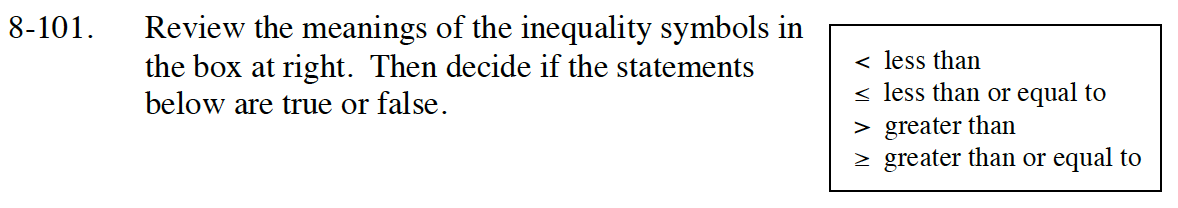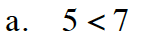Is 5 less than 7?

True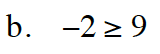Is −2 greater than or equal to 9?

False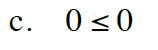Is 0 less than or equal to 0?

True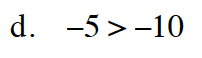Is −5 greater than −10?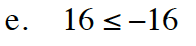Is 16 less than or equal to −16?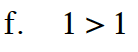Is 1 greater than 1?